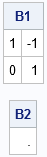Did you know that a SAS/IML function can recover from a run-time error? You can specify how to handle run-time errors by using a programming technique that is similar to the modern "try-catch" technique, although the SAS/IML technique is an older implementation.

### Preventing errors versus handling errors

In general, SAS/IML programmers should try to detect potential problems and prevent errors from occurring. For example, before you compute the square root of a number, you should test whether the number is greater than or equal to zero. If not, you can handle the bad input. By testing the input value before you call the SQRT function, you prevent a run-time error.

However, sometimes you don't know whether a computation will fail until you attempt it. Other times, the test to determine whether an error will occur is very expensive, and it is cheaper to attempt the computation and handle the error if it occurs.

A canonical example is computing the inverse of a square matrix. It is difficult to test whether a given matrix is numerically singular. You can compute the rank of the matrix or the condition number of the matrix, but both computations are expensive because they rely on the SVD or eigenvalue decomposition. Cheaper methods include computing a determinant or using Gaussian elimination, but these methods can be numerically unstable and are not good indicators of whether a matrix is numerically singular.

### Run-time error in SAS/IML modules

To understand how to handle run-time errors in SAS/IML modules, recall the following facts about how to use the execution queue in SAS/IML modules.

In summary, when a run-time error occurs in a module, the module pauses and waits for additional commands. However, if there are statements in the execution queue, those statements will be executed.

You can use these facts to handle errors. At the top of the module, use the PUSH statement to add error-handling statements into the execution queue. If an error occurs, the module pauses, sees that there are statements in the queue, and executes those statements. If one of the statements contains a RESUME statement, the module resumes execution.

### Push, pause, execute, and resume

Let's apply these ideas to computing the inverse of a square matrix. The following SAS/IML function attempts to compute the inverse of the input matrix. If the matrix is singular, the computation fails and the module handles that error. The main steps of the modules are as follows:

1. Define the statements that will be executed in the event of an error. The last executable statement should be the RESUME statement. (Technical point: The RESUME statement is only valid when the module is paused. Surround the statements with IF-THEN logic to prevent the error-handling statements from running when the module exits normally.)
2. Push these statements into the execution queue.
3. If an error occurs, the statements in the queue will be executed. The RESUME statement clears the error and resumes execution of the module. (If no error occurs, the queue is flushed after the RETURN statement.)
```proc iml; /* If the matrix is not invertible, return a missing value. Otherwise, return the inverse. */ start InvEx(A); errFlag = 1; /* set flag. Assume we will get an error :-) */ on_error = "if errFlag then do; AInv = .; resume; end;"; call push(on_error); /* PUSH code that will be executed if an error occurs */ AInv = inv(A); /* if error, AInv set to missing and function resumes */ errFlag = 0; /* remove flag for normal exit from module */ return ( AInv ); finish;   A1 = {1 1, 0 1}; B1 = InvEx(A1); A2 = {1 1, 1 1}; B2 = InvEx(A2);   print B1, B2;```The function is called first with a nonsingular matrix, A1. The INV function does not fail, so the module exits normally. When the module exits, it flushes the execution queue. Because ErrFlag=0, the pushed statements have no effect. B1 is equal to the inverse of A1.

The function is called next with a singular matrix, A2. The INV function encounters an error and the module pauses. When the module pauses, it executes the statements in the queue. Because ErrFlag=1, the statements set AINV to a missing value and resume the module execution. The module exits normally and the B2 value is set to missing.

### Summary

In summary, you can implement statements that handle run-time errors in SAS/IML modules. The key is to push error-handling code into a queue. The error-handling statements should include a RESUME statement. If the module pauses due to an error, the statements in the queue are executed and the module resumes execution. Be aware that the queue is flushed when the module exits normally, so use a flag variable and IF-THEN logic to control how the statements behave when there is no error.

The post Catch run-time errors in SAS/IML programs appeared first on The DO Loop.